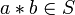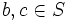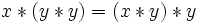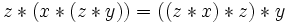# Associative binary operation

This article defines a property of binary operations (and hence, of magmas)

## Definition

### Definition in infix notation

Let$S$ be a set and$*$ be a binary operation on$S$ (viz,$*$ is a map$S \times S \to S$), making$(S,*)$ a magma. We denote$*$ using infix notation, so that its application to$x,y \in S$ is denoted$x * y$. Then,$*$ is said to be associative if, for every$a, b, c$ in$S$, the following identity holds:$(a * b) * c = a * (b * c)$

where equality holds as elements of$S$.

Note that$a,b,c$ are allowed to be equal or distinct. In particular, the above holds when all are equal, all are distinct, or two are equal and the third distinct.

Detailed explanation of expressions and their interpretation: The left side expression$(a * b) * c$ is termed the left associated expression for$a,b,c$ and is interpreted and evaluated as follows. We first consider$a * b$. Since$a,b \in S$, we have$a * b \in S$. We now consider the elements$a * b,c \in S$. Since both of these are in$S$,$(a * b) * c \in S$.

The right side expression$a * (b * c)$ is termed the right associated expression for$a,b,c$ and is interpreted and evaluated as follows. Since$b,c \in S$, we have$b * c \in S$. We consider consider the elements$a, b * c \in S$. Since both of these are in$S$,$a * (b * c) \in S$.

If, for a given$a, b, c$, the left associated expression and the right associated expression are equal,$a, b, c$ are said to associate. Associativity basically says that every ordered triple of elements associates.

### Definition in usual function notation

Let$S$ be a set and$f: S \times S \to S$ be a binary operation. We say that$f$ is associative if it satisfies the following for all$a,b,c \in S$:$f(f(a,b),c) = f(a,f(b,c))$

We see that the condition feels a lot less intuitive in function notation than with the infix notation, which is why infix notation is generally preferred for describing associativity in the context of binary operations.

### Related term

A set equipped with an associative binary operation is termed a semigroup. If, further, there is a neutral element (identity element) for the associative binary operation, the set is termed a monoid.

## Numerical invariants

The associative law is typically viewed as a universally quantified identity. In this context, we discuss some invariants that can be associated with the identity.

Invariant Value Significance
minimum number of variables in terms of which the identity can be expressed 3 A magma is associative if and only if the submagma generated by any$3$ elements is associative; nothing smaller than$3$ works
if homogeneous, then total degree 3 The associative law is homogeneous of degree three, i.e., all expressions are products of length three. This allows us to use the techniques of operad theory. For more, see associativity operad.
degree of the identity in each variable 1 when this identity is viewed for the multiplicative operation of a ring or algebra, it is a linear identity in each variable. In particular, the set of values for each variable such that the identity holds for all values of the other variables is an additive subgroup.

## Facts

### Parenthesization can be dropped

For full proof, refer: Associative implies generalized associative

When a binary operation is associative, it turns out that we can drop parenthesization from products of many elements. That is, given an expression of the form:$a_1 * a_2 ... * a_n$

any choice of bracketing will give the same result.

The result is proved by induction, with the base case ($n = 3$) following from the definition of associativity.

As an illustration, suppose we want to show that:$a_1 * (a_2 * (a_3 * a_4)) = ((a_1 * a_2) * a_3) * a_4$

Then, we apply associativity in a chain:$a_1 * (a_2 * (a_3 * a_4)) = a_1 * ((a_2 * a_3) * a_4) = (a_1 * (a_2 * a_3)) * a_4 = ((a_1 * a_2) * a_3) * a_4$

For this reason, we always use infix operator symbols for associative binary operations, and often even drop the operator symbol, so that the expression$a_1 * a_2 ... * a_n$ is just written as:$a_1a_2 \dots a_n$.

Also, the re-parenthesization identities (i.e., all identities that are special cases of generalized associativity) are the only identities that can be proved using associativity.

### Associativity pentagon

Further information: Associativity pentagon

The associativity pentagon is a pentagon whose vertices are the five different ways of associating a product of length four, with an edge between two vertices if moving from one to the other requires a single application of the associative law. This is a cyclic pentagon. The associativity pentagon is significant because, loosely, it generates all relations between the different ways of applying the associativity law to re-parenthesize expressions. It also helps to prove results about the set of left-associative, middle-associative, and right-associative elements. It is also related to the associator identity.

### Power structure

Further information: power-associative magma

In the presence of associativity, it is possible to unambiguously define positive powers of any element. Explicitly,$x^n$ is the$n$-fold product$x * x * \dots * x$. The powers satisfy the usual laws of powers:$x^m * x^n = x^{m + n}$ and$(x^m)^n = x^{mn}$ for all$m,n \in \mathbb{N}$. Note that this also implies that all powers of$x$ commute with each other.

Note that to define powers, we do not actually need global associativity, but only power-associativity: the submagma generated by any single element must be associative.

### Verification of associativity for a ring

Suppose$R$ is a commutative unital ring and$A$ is a (possibly associative, possibly non-associative) algebra over$R$. In other words,$A$ is a$R$-module and$*: A \times A \to A$ is a (possibly associative, possibly non-associative) binary operation.

Note first that in order to verify the associativity of multiplication globally, it suffices to verify associativity on a generating set for the additive group of$A$ as a$R$-module. This is because the associator function is$R$-linear in each input:$a(x,y,z) = ((x * y) * z) - (x * (y * z))$

In the special case that$A$ is freely generated as a$R$-module, the following test works for associativity: If$e_i, i \in I$ form a freely generating set of$A$ as a$R$-module, and$\lambda_{ij}^k$ denote the structure constants, then associativity is the following identity for all$i,j,k,l \in I$:$\sum_{m \in I} \lambda_{ij}^m\lambda_{mk}^l = \sum_{m \in I} \lambda_{im}^l \lambda_{jk}^m$

### Associator on a non-associative ring and the associator identity

Further information: associator on a non-associative ring

For a non-associative ring$R$ with multiplication$*$, we can define the associator$a:R \times R \times R \to R$ as:$a(x,y,z) = ((x * y) * z) - (x * (y * z))$

This is linear in each of its variables.

## Related element properties

### Left-associative element

An element is said to be left-associative or left nuclear with respect to a binary operation if any ordered triple starting with that element associates.

The set of left-associative elements in any magma is a subsemigroup called the left nucleus. If the magma contains a neutral element, it is a submonoid.

For full proof, refer: Left-associative elements of magma form submagma

### Middle-associative element

An element is said to be middle-associative or middle nuclear with respect to a binary operation if any ordered triple with that element in the middle, associates.

The set of middle-associative elements in any magma is a subsemigroup called the middle nucleus. and if the magma contains a neutral element, it is a submonoid.

For full proof, refer: Middle-associative elements of magma form submagma

### Right-associative element

An element is said to be right-associative with respect to a binary operation if any ordered triple ending with that element associates.

The set of right associative elements in any magma is a subsemigroup called the right nucleus. If the magma contains a neutral element, it is a submonoid.

For full proof, refer: Right-associative elements of magma form submagma

### Associative element

Further information: associative element

An element is said to be associative if it is left, middle and right associative. The set of associative elements forms a submagma (which contains the neutral element if it exists) termed the associative center (or sometimes, the nucleus) of the magma.

## Weaker identities

### Identities obtained directly by duplicating variables

Algebraic formulation of identity Name of identity Name of magma satisfying identity Name of non-associative ring satisfying identity$\! x * (x * y) = (x * x) * y$ left-alternativity left-alternative magma left-alternative ring$\! x * (y * y) = (x * y) * y$ right-alternativity right-alternative magma right-alternative ring$\! x * (y * x) = (x * y) * x$ flexibility flexible magma flexible ring$\! x * (x * x) = (x * x) * x$  ? magma in which cubes are well-defined ring in which cubes are well-defined

### Identities obtained from generalized associativity by duplicating variables

We here discuss identities with products of length at most four:

Algebraic formulation of identity Name of identity Name of magma satisfying identity Name of non-associative ring satisfying identity$\! (x * y) * (x * x) = x * (y * (x * x))$ Jordan's identity  ? (if we also assume commutativity, then Jordan magma)  ? (if we also assume commutativity, then Jordan ring)$\! z * (x * (z * y)) = ((z * x) * z) * y$ One of Moufang's identities  ?  ?$\! x * (z * (y * z)) = ((x * z) * y) * z$ One of Moufang's identities  ?  ?$\! (z * x) * (y * z) = (z * (x * y)) * z$ One of Moufang's identities  ?  ?